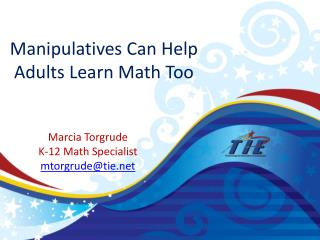DownloadDownload PresentationManipulatives Can Help Adults Learn Math Too

# Manipulatives Can Help Adults Learn Math Too

Download Presentation## Manipulatives Can Help Adults Learn Math Too

- - - - - - - - - - - - - - - - - - - - - - - - - - - E N D - - - - - - - - - - - - - - - - - - - - - - - - - - -
##### Presentation Transcript

1. Manipulatives Can Help Adults Learn Math Too Marcia Torgrude K-12 Math Specialist mtorgrude@tie.net

2. Number Sense “Being able to handle numbers comfortably and competently is important to adults as parents, workers and community members. This competence relies upon having developed a kind of "number sense" about whole numbers, money, fractions, decimals, and percentages. Number sense includes calculation skills with numbers as well as a sense of number and operation and an ability to appropriately use estimation, mental math, computation, calculators or other tools.” The Massachusetts Mathematics Curriculum Framework http://shell04.theworld.com/std/anpn//frame5.html

3. Outcomes • Mental Math – for daily life skills • Using manipulativesat any age to gain an understanding of numbers • Multiplication/Division strategies • Develop Fraction sense • Visualize decimals for understanding • Build Algebraic understanding hands-on

4. Mental Math If I earn \$415 per week. How much will I earn in 20 weeks? No paper or pencil – how could we solve this mentally? • Estimation • Breaking numbers apart • Compensation

5. Manipulatives for Multiplication • Base ten blocks • Grid/Array model • Compare to traditional algorithm What are your thoughts?

6. Manipulatives for Division • Grid • Friendly number or partial quotient division • Compare to traditional algorithm What are your thoughts? Why is this important for adult learners?

7. Working with Fractions • Parts of a whole • Pattern Blocks • Hands on • Patch Tool – Illuminations - http://illuminations.nctm.org/ActivityDetail.aspx?ID=27 • Cuisinaire Rods

8. Working with Fractions • Equivalent Fractions • http://illuminations.nctm.org/ActivityDetail.aspx?id=18

9. Working with Fractions • Adding Fractions • Pattern Blocks • Cuisinaire rods

10. Working with Fractions • Making Sense of Multiplying Fractions • You have two thirds of an enormous brownie and decide to eat one fourth of the two thirds. How much of the brownie are you eating? • Making Sense of Dividing fractions • I have 3 ½ pounds of hamburger. I want to make quarter pound burgers. How many can I make?

11. Working with Decimals • Base ten blocks to build decimal number sense • Graph paper and decimals

12. Working with Integers • Building understanding of positive and negative numbers • Adding and Subtracting positive and negative • Use of Charge Model • Use of the linear model

13. Algebraic Equations • Algebra Tiles to develop an understanding of algebraic expressions and equations • Adding/Subtracting • Multiplying • Using the Array or Grid model • Connecting to the traditional algorithms

14. Working with Algebra TilesExpressions • Build 3x +2 + (-4x) +(-5) • Simplify this. • Now try: • 5x-9-2-3x

15. Working with Algebra TilesAddition/Subtraction • Build 2x2 + 3x + 5 • Now add x2 – 2x • Solve • Now try: 2x2 +4x – 5 – (x2 +2x -3)

16. Working with Algebra TilesMultiplication • Build (x+3) (x- 2) • Solve • Now try: (-2x + 2)(x – 3)

17. Working with Algebra TilesFactoring • Create an array for x2 + 5x + 6 • What are the factors? • Now try: x2 – 7x + 12 • http://mathbits.com/mathbits/AlgebraTiles/AlgebraTilesMathBitsNew07ImpFree.html

18. Open Discussion • What understanding did you build today? • How might you use the manipulatives with adult learners? • What questions do you have?